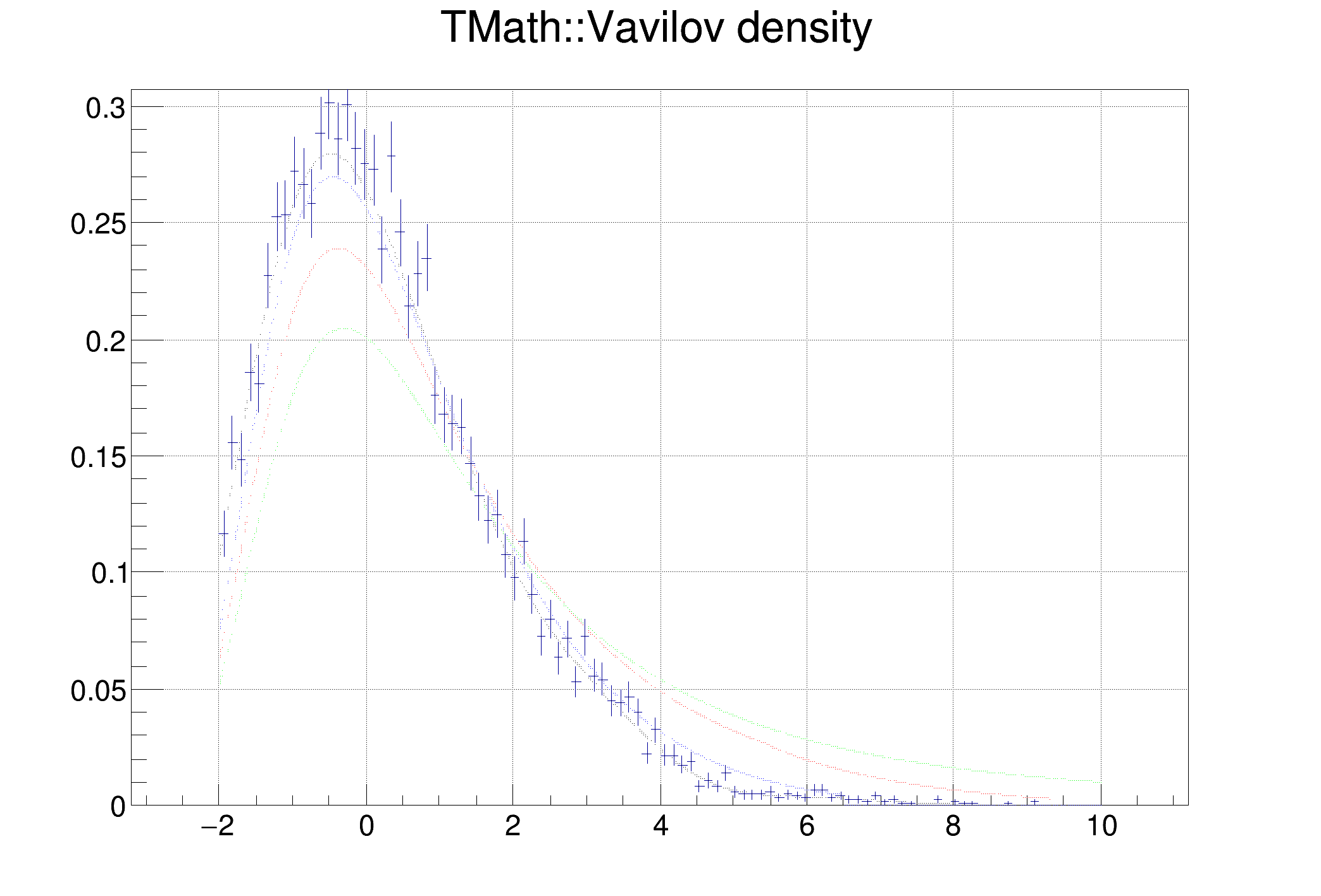ROOT   Reference Guide
Searching...
No Matches
vavilov.C File Reference

## Detailed Description#include "TMath.h"
#include "TCanvas.h"
#include "TRandom.h"
#include "TGraph.h"
void vavilov()
{
Int_t n = 1000;
Double_t *xvalues = new Double_t[n];
Double_t *yvalues1 = new Double_t[n];
Double_t *yvalues2 = new Double_t[n];
Double_t *yvalues3 = new Double_t[n];
Double_t *yvalues4 = new Double_t[n];
for (Int_t i=0; i<n; i++) {
xvalues[i] = r.Uniform(-2, 10);
yvalues1[i] = TMath::Vavilov(xvalues[i], 0.3, 0.5);
yvalues2[i] = TMath::Vavilov(xvalues[i], 0.15, 0.5);
yvalues3[i] = TMath::Vavilov(xvalues[i], 0.25, 0.5);
yvalues4[i] = TMath::Vavilov(xvalues[i], 0.05, 0.5);
}
TCanvas *c1 = new TCanvas("c1", "Vavilov density");
c1->SetGrid();
c1->SetHighLightColor(19);
TGraph *gr1 = new TGraph(n, xvalues, yvalues1);
TGraph *gr2 = new TGraph(n, xvalues, yvalues2);
TGraph *gr3 = new TGraph(n, xvalues, yvalues3);
TGraph *gr4 = new TGraph(n, xvalues, yvalues4);
gr1->SetTitle("TMath::Vavilov density");
gr1->Draw("ap");
gr2->Draw("psame");
gr3->Draw("psame");
gr4->Draw("psame");
TF1 *f1 = new TF1("f1", "TMath::Vavilov(x, 0.3, 0.5)", -2, 10);
TH1F *hist = new TH1F("vavilov", "vavilov", 100, -2, 10);
for (int i=0; i<10000; i++) {
hist->Fill(f1->GetRandom());
}
hist->Scale(1/1200.);
hist->Draw("same");
}
ROOT::R::TRInterface & r
Definition Object.C:4
int Int_t
Definition RtypesCore.h:45
double Double_t
Definition RtypesCore.h:59
@ kRed
Definition Rtypes.h:66
@ kGreen
Definition Rtypes.h:66
@ kBlue
Definition Rtypes.h:66
virtual void SetMarkerColor(Color_t mcolor=1)
Set the marker color.
Definition TAttMarker.h:38
The Canvas class.
Definition TCanvas.h:23
1-Dim function class
Definition TF1.h:213
virtual Double_t GetRandom(TRandom *rng=nullptr, Option_t *opt=nullptr)
Return a random number following this function shape.
Definition TF1.cxx:2180
A TGraph is an object made of two arrays X and Y with npoints each.
Definition TGraph.h:41
virtual void SetTitle(const char *title="")
Change (i.e.
Definition TGraph.cxx:2339
virtual void Draw(Option_t *chopt="")
Draw this graph with its current attributes.
Definition TGraph.cxx:769
1-D histogram with a float per channel (see TH1 documentation)}
Definition TH1.h:575
virtual Int_t Fill(Double_t x)
Increment bin with abscissa X by 1.
Definition TH1.cxx:3350
virtual void Draw(Option_t *option="")
Draw this histogram with options.
Definition TH1.cxx:3073
virtual void Scale(Double_t c1=1, Option_t *option="")
Multiply this histogram by a constant c1.
Definition TH1.cxx:6564
This is the base class for the ROOT Random number generators.
Definition TRandom.h:27
return c1
Definition legend1.C:41
const Int_t n
Definition legend1.C:16
TF1 * f1
Definition legend1.C:11
Double_t Vavilov(Double_t x, Double_t kappa, Double_t beta2)
Returns the value of the Vavilov density function.
Definition TMath.cxx:2734

Definition in file vavilov.C.